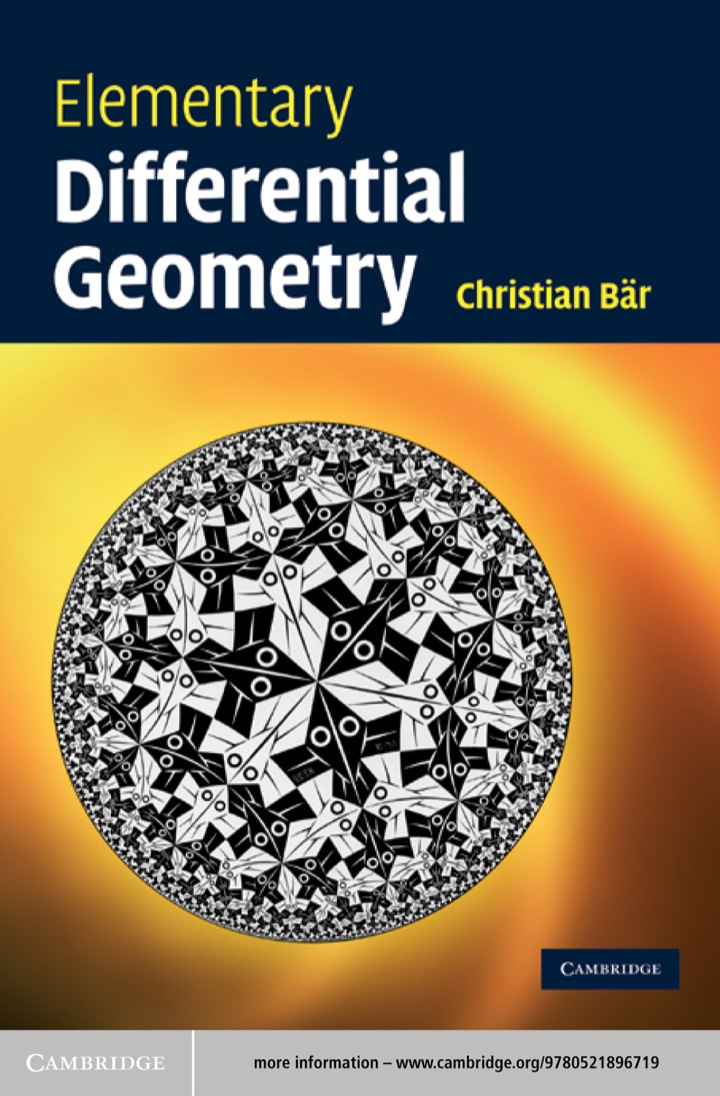# Elementary Differential Geometry

## Elementary Differential Geometry

By Christian Bär

Expires on May 15th, 2022
\$50

Publisher List Price: \$0.00

The link between the physical world and its visualization is geometry. This easy-to-read, generously illustrated textbook presents an elementary introduction to differential geometry with emphasis on geometric results. Avoiding formalism as much as possible, the author harnesses basic mathematical skills in analysis and linear algebra to solve interesting geometric problems, which prepare students for more advanced study in mathematics and other scientific fields such as physics and computer science. The wide range of topics includes curve theory, a detailed study of surfaces, curvature, variation of area and minimal surfaces, geodesics, spherical and hyperbolic geometry, the divergence theorem, triangulations, and the Gauss–Bonnet theorem. The section on cartography demonstrates the concrete importance of elementary differential geometry in applications. Clearly developed arguments and proofs, colour illustrations, and over 100 exercises and solutions make this book ideal for courses and self-study. The only prerequisites are one year of undergraduate calculus and linear algebra.

Subject: Mathematics & Statistics -> Post-Calculus -> Topology1st edition
Publisher: Cambridge University Press 5/6/10
Imprint: Cambridge University Press
Language: English

ISBN 10: 1139637835
ISBN 13: 9781139637831
Print ISBN: 9780521721493

Live Chats